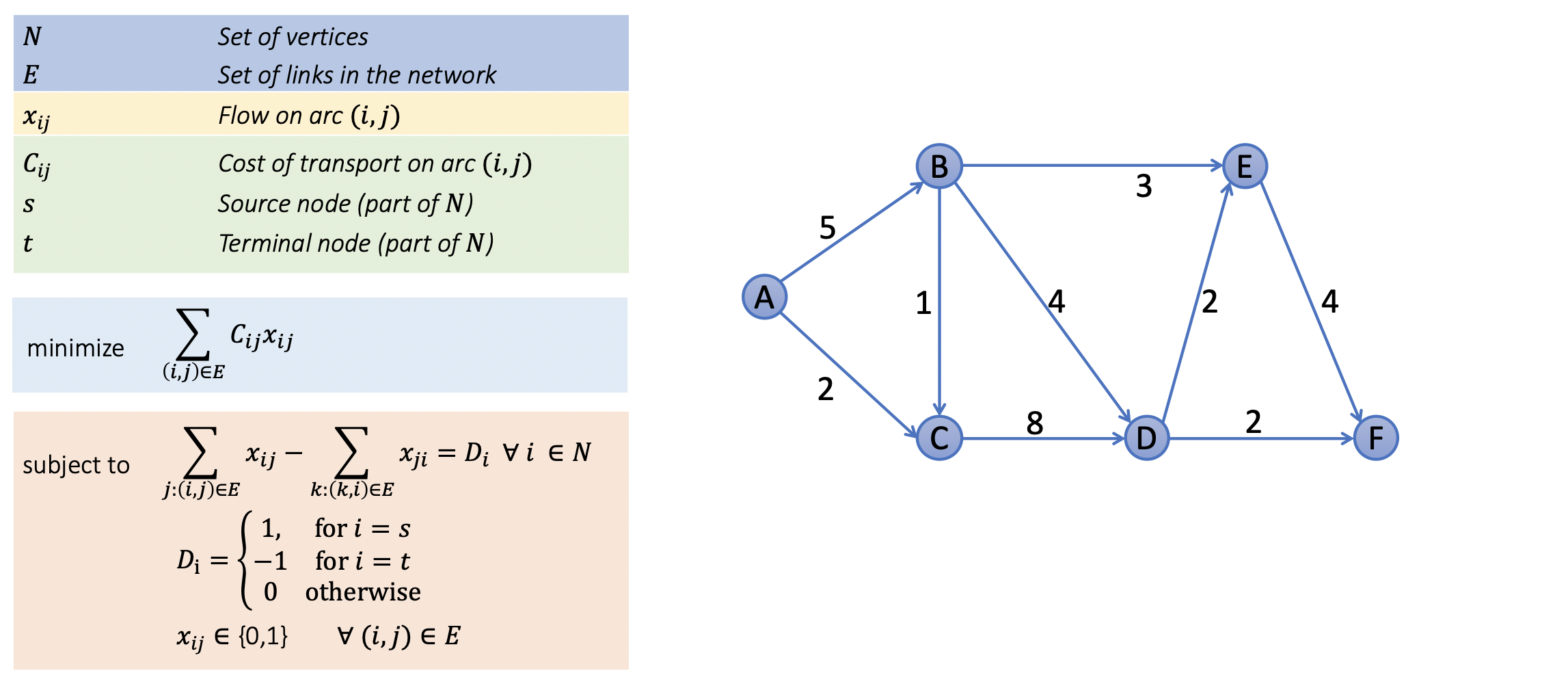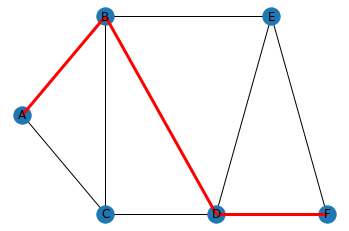# Notebook 3.6 - Shortest paths using PuLP¶In :
# Setting up

!pip install pulp --progress-bar off

from pulp import *

prob = LpProblem('prob', LpMinimize)

Requirement already satisfied: pulp in /Users/pan/anaconda3/lib/python3.7/site-packages (2.0)
Requirement already satisfied: pyparsing>=2.0.1 in /Users/pan/anaconda3/lib/python3.7/site-packages (from pulp) (2.4.7)

In :
# Defining problem instance

N = ['A', 'B', 'C', 'D', 'E', 'F']

C = {'A': {'B': 5, 'C': 2},
'B': {'C': 1, 'D': 4, 'E': 3},
'C': {'D': 8},
'D': {'E': 2, 'F': 2},
'E': {'F': 4}}

D = {node: 1 if node is 'A' else -1 if node is 'F' else 0 for node in N}

In :
# Preparing "tables"

E = [(i,j) for i in N for j in N if i in C.keys() if j in C[i].keys()]

In :
# Declaring decision variables.

x = LpVariable.dicts('x', E,  lowBound = 0, upBound = 1, cat = LpInteger)


PuLP does not have a built-in type for Boolean decision variables. To overcome this limitation we will be declaring our $x_{ij}$ variables as integers (using LpInteger), while constraining them to take values between 0 and 1.

In :
# Declaring objectives

prob += lpSum([C[i][j]*x[i,j] for (i,j) in E])

for i in N:
prob += (lpSum([x[i,j] for j in N if (i,j) in E])
- lpSum([x[k,i] for k in N if (k,i) in E])) == D[i]

In :
# Solving problem

status = prob.solve()

print(f'STATUS\n{LpStatus[status]}\n')

print('SOLUTION')
for v in prob.variables():
print(v.name, '=', v.varValue)

STATUS
Optimal

SOLUTION
x_('A',_'B') = 1.0
x_('A',_'C') = 0.0
x_('B',_'C') = 0.0
x_('B',_'D') = 1.0
x_('B',_'E') = 0.0
x_('C',_'D') = 0.0
x_('D',_'E') = 0.0
x_('D',_'F') = 1.0
x_('E',_'F') = 0.0

In :
import networkx as nx
from matplotlib import pyplot, collections
position = {'A': [0,0.5], 'B': [3,1], 'C': [3,0],'D': [7,0], 'E': [9, 1], 'F': [11,0]}
flow = [v.varValue*3 for v in prob.variables()]
G = nx.Graph()

<matplotlib.collections.LineCollection at 0x7f9e79c983d0>